Name:    2nd Grade Math Proficient Test 6

Multiple Choice
Identify the choice that best completes the statement or answers the question.

1.

Which figure is a right angle?
 a.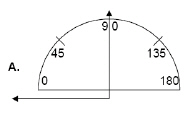b.c.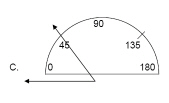d.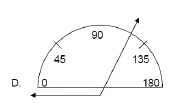e.2.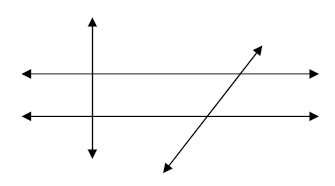How many angles are right angles?
 a. 2 d. 16 b. 8 e. 6 c. 4

3.

How many sets of parallel lines are in the figure?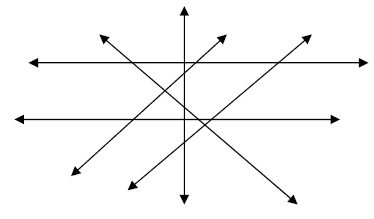a. 1 d. 5 b. 0 e. 3 c. 2

4.

Which figure shows a diagonal line?
 a.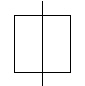d.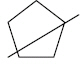b.e.c.5.

Measurement – Area, Perimeter, Circumference – RIT 181 - 190a. 10 d. 12 b. 11 e. 8 c. 9

6.a. 18 square units d. 16 square units b. 9 square units e. 5 square units c. 20 square units

7.

What is the perimeter?a. 2 inches d. 12 inches b. 8 inches e. 6 inches c. 10 inches

8.

Measurement – Length, Height, Volume – RIT 181 – 190a. 5g d. 6g b. 2g e. 7g c. 1g

9.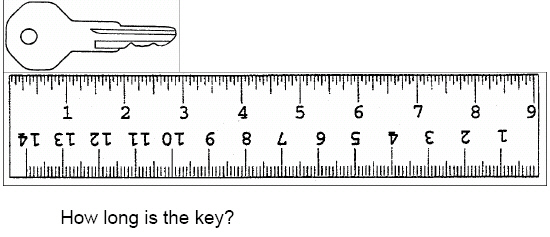a. 3 inches d. 5 inches b. 4 inches e. 1 inch c. 2 inches

10.

Measurement – Money – RIT 181 – 190
Which collection of coins has pennies?
 a.b.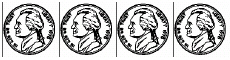c.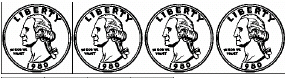d.e.11.a. 1¢ d. 50¢ b. 5¢ e. 10¢ c. 25¢

12.

3 dollars, 1 quarter, and 1 dime equals:
 a. \$3.35 d. \$3.25 b. \$1.35 e. \$3.10 c. \$2.35

13.a.b.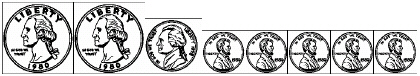c.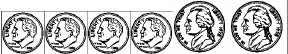d.e.14.

A candy bar cost 35¢. Mary gave the clerk \$1.00. Which shows the correct
change given to her?
 a.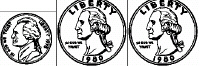d.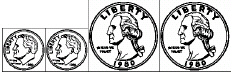b.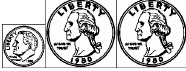e.c.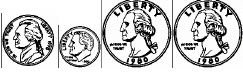15.

\$7.83
-\$4.95
 a. \$3.12 d. \$2.88 b. \$3.88 e. \$2.28 c. \$12.78